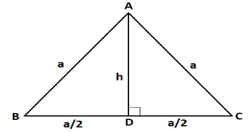# Area of Equilateral Triangle Formula

## How to Find the Area of an Equilateral Triangle - Definitions & Examples

An equilateral triangle is one in which all three sides are equal in length. Consequently, each of its three interior angles measure a third of $180^\circ$, which is $60^\circ$ each. One of the most interesting and useful properties of an equilateral triangle is that its altitude, angle bisector and median from any of its vertices are coincident (they are the same line segment). This can be proved using the concept of congruence of triangles. Consider the following equilateral triangle ABC whose side length is a units. Since AD is the median to BC, BD = DC = BC/2 = a/2 units.Since AD is also the perpendicular to BC, we can use the Pythagorean theorem to determine its length (which is the height of the triangle corresponding to the side BC).
$A{C^2} = A{D^2} + D{C^2} \Rightarrow A{D^2} = A{C^2} - D{C^2} = {a^2} - {\left( {\frac{a}{2}} \right)^2} = {a^2} - \frac{{{a^4}}}{4} = \frac{{3{a^2}}}{4}\, \Rightarrow AD = h = \frac{{\sqrt 3 a}}{2}$
area$\left( {\Delta ABC} \right) = \frac{1}{2}bh = \frac{1}{2} \times BC \times AD = \frac{1}{2} \times a \times h = \frac{1}{2} \times a \times \frac{{\sqrt 3 a}}{2} = \frac{{\sqrt 3 }}{4}{a^2}$
Note that we can derive this formula using Heron’s formula as well.
$s = \frac{{\left( {a + b + c} \right)}}{2} = \frac{{3a}}{2}$
area$\left( {\Delta ABC} \right) = \sqrt {s\left( {s - a} \right)\left( {s - b} \right)\left( {s - b} \right)}$
$= \sqrt {\left( {\frac{{3a}}{2}} \right)\left( {\frac{{3a}}{2} - a} \right)\left( {\frac{{3a}}{2} - a} \right)\left( {\frac{{3a}}{2} - a} \right)}$
$\sqrt {\left( {\frac{{3a}}{2}} \right)\left( {\frac{a}{2}} \right)\left( {\frac{a}{2}} \right)\left( {\frac{a}{2}} \right)}$
$= \sqrt {\frac{{3{a^4}}}{{16}}}$
$= \frac{{\sqrt 3 }}{4}{a^2}$
Let’s look at an example to see how to use this formula.

Question: Find the area of an equilateral triangle whose altitude is $3\sqrt 3 \,cm$.
Solution:
$h = 3\sqrt 3 \,cm$
$h = \frac{{\sqrt 3 a}}{2}\, \Rightarrow \frac{{\sqrt 3 a}}{2} = 3\sqrt 3 \Rightarrow a = 6\,cm$
area $= \frac{{\sqrt 3 }}{4}{a^2} = \frac{{\sqrt 3 }}{4}{6^2} = 9\sqrt 3 \,c{m^2}$
Why don’t you try to solving a problem for practice?

Question: Find the area of an equilateral triangle whose perimeter is 12 cm.
Options:
(a) $2\sqrt 3 \,c{m^2}$
(b) $4\sqrt 3 \,c{m^2}$
(c) $6\sqrt 3 \,c{m^2}$
(d) $8\sqrt 3 \,c{m^2}$
Perimeter $= 3a = 12\,\,cm\,\,\, \Rightarrow a = 4\,\,cm$
area $= \frac{{\sqrt 3 }}{4}{a^2} = \frac{{\sqrt 3 }}{4}{4^2} = 4\sqrt 3 \,\,c{m^2}$